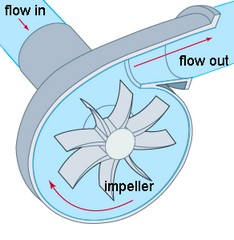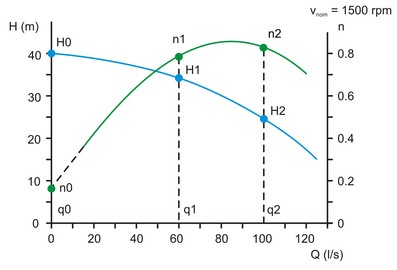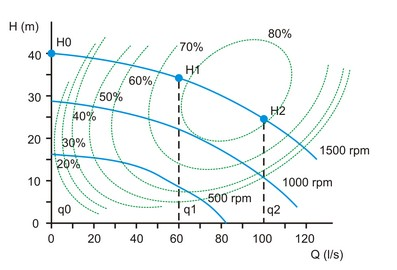﻿ 20-sim webhelp > Library > Iconic Diagrams > Hydraulics > Pumps > CentrifugalPump

# CentrifugalPump

## Library

Iconic Diagrams\Hydraulics\Pumps

## Use

Domains: Continuous. Size: 1-D. Kind: Iconic Diagrams (Hydraulics).

Description

A centrifugal pump converts the input power of a rotating shaft into kinetic energy of a the liquid by accelerating the liquid through an impeller.The pump is characterized by two curves:

 1 The head (H) as function of the volume flow at a preset speed.
 2 The efficiency (n) of the pump as a function of the fluid flow at a present speed. The efficiency is defined as the flow output power divided by rotational input power.The pump curves are approximated by two second order polynomials:

H = ha*q2 + hb*q + hc

n = na*q2 + nb*q + nc

To fit the polynomial curves, the heads and efficiencies have to be specified at three different flows. The first flow (q0) is zero. The other two flows (q1 and q2) can be chosen arbitrary.

Note: some pump flows extrapolate the efficiency curves to zero at zero fluid flow. This would mean that at a zero fluid flow no power can be transmitted and the pump can never start! Therefore in this model always use an efficiency that is non-zero at zero flow.Some  pump characteristics give multiple input speeds. This model automatically takes into account for varying input speeds of the input axis by transforming the  pump curve using the affinity rules.

Ha = Hb*(vb/va)2

Qa = Qb*(vb/va)

Some pump characteristics give the efficiencies as regions. You have then have to estimate the efficiencies at the chosen flows q0, q1 and q2.

## Interface

 Ports Description p_t p_a p_rot inlet port, tank (hydraulic) outlet port (hydraulic) axis (rotation) Causality velocity out p_rot Input phi Volume flow [m3/s] Parameters q1 q2 H0 H1 H2 n0 n1 n2 v_nom rho flow 1 (choose an arbitrary point between zero and nominal) flow 2 (nominal flow) head at zero flow head at flow 1 head at flow 2 (nominal flow) efficiency (0 < n < 1) at zero flow efficiency (0 < n < 1) at flow 1 efficiency (0 < n < 1) at flow 2 (nominal flow) nominal speed of the pump fluid density